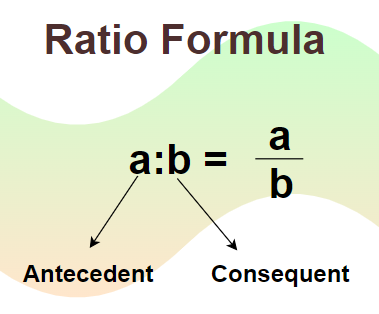Open In App

# Ratio Formula

Ratios are an important concept in mathematics that is used to solve various numerical problems. It is another way of representing fractions and we define ratio using the ‘:‘ symbol. It is defined as the amount of an object contained in another object. Suppose we have two numbers ‘a’ and ‘b’ then we define the ratio between a and b as,

a:b and is read as “a ratio b” and its value is given as, a:b = a/b

As in fractions, we say ‘a’ in a/b the numerator, and ‘b’ the denominator. Similarly, in a:b we say ‘a‘ the Antecedent and ‘b‘ the Consequent.

Now, let us learn about what is ratio, ratio formulas including examples, and others in detail in this article.

## Ratio Definition

We define ratios as the comparison between two quantities of similar units. Ratios tell us how much one quantity is present in another quantity. We define the ratio as the way of expressing the mathematical concept of comparing two quantities. Suppose in a class of 35 students we have 20 girls and 15 boys then the ratio between girls and boys in that class is, 20:15 further simplifying we can say that the ratio between girls and boys is 4:3 which implies for every 4 girls in the class we have 3 boys.

## What is Ratio Formula?

As we already know that ratios are used to define the relationship between two similar quantities and it explains the amount of the first quantity contained in another quantity. Ratios are represented as, a:b and are read as “a is to b” but solving the ratio uses the ratio formulas which convert the ratio into fractions and then it is easily solved. The ratio formula which converts the ratio into a fraction is,

a:b = a/b

The image added below shows the ratio formula,It is clear from the above formula that if a and b are individual quantities then the total quantity is given using the formula (a+b).

## How to Calculate Ratios?

As we know that the fraction a/b is represented as the ratio a:b and we can easily calculate the ratio by finding the suitable fraction and then simplify it to get the simplest form.

We can understand this with the help of the example discussed below:

Example: Find the ratio of the marks obtained by Vihan in mathematics and science if he scores 68 marks in mathematics and 74 marks in science.

Solution:

We can represent the ratio of marks in Mathematics and Science as,

Mathematics:Science = 68:74

This can be changed into fractions using the ratio formula,

Mathematics:Science = 68:74 = 68/74

simplifying,

Mathematics:Science = 68/74 = 34/37

Thus, we can simplify that ratio as,

Mathematics:Science = 34:37

## Examples on Ratio Formula

Example 1: In a class of 80 students, there are 45 girls and the remaining are boys. Find the ratio of the total number of boys to the number of girls.

Solution:

Total Number of Students in the class = 80

Number of Girls = 45

Number of Boys = Total Number of Students – Number of Girls
= 80 – 45 = 35

Ratio of Number of Boys and Number of Girls,

Number of Boys: Number of Girls = 45:35

Using the Ratio Formula,

45:35 = 45/35

= 9/7

Thus, the ratio of the number of boys and the number of girls is 9:7

Example 2: If the ratio of two supplementary angles is 2:3. Find the angles.

Solution:

Given,

Ratio of the supplementry angle = 2:3

Let the angle be 2x and 3x

Now, we know that supplementry angles are the angles whose sum is 180 degrees. Then,

2x + 3x = 180°

5x = 180°

x = 36°

Now,

First Angle = 2x = 2×36 = 72°

Second Angle = 3x = 3×36 = 108°

Thus, the required angles are 72° and 108°

Example 3: A basket consists of 16 oranges and 12 mangoes. Find the ratio of orange to mango in the basket.

Solution:

Given,

• Number of Oranges = 16
• Number of Mangoes = 12

Then,

Ratio of Orange to Mango = 16:12 = 16/12

Simplifying further,

16/12 = 4/3

Thus, the ratio of Orange to Mango is 4/3 or 4:3

Example 4: If the ratio of x and y is 3:5 and x = 21, then find the value of y.

Solution:

Given:

x:y = 3:5

x = 21

Using the Ratio Formula,

x:y = 3:5

x/y = 3/5

21/y = 3/5

y = (21×5)/3

y = 35

Thus, the value of y is 35

## FAQs on Ratio Formula

### Q1: What is Ratio?

Ratios are a way of representing similar quantities. We define ratio as the comparison between two quantities such that it tells us how much of one quantity is present in the other quantity.

### Q2: How to Find the Ratio using the Ratio Formula?

The ratio can be easily found using the ratio formula by following the steps discussed below,

Step 1: Mark the quantities for which we have to find the ratio say A and B.

Step 2: Find the value of the fraction A/B to find the ratio A is to B.

Step 3: Find the simplest form of A/B say A/B = a/b.

Step 4: Using the ratio formula we get the required ratio as,

A:B = a:b

### Q3: What is Ratio Formula?

The ratio formula is the basic formula which converts the ratio into fraction form and vice-versa. The ratio formula is,

a:b = a/b

### Q4: How to Find the Simplest form of Ratio using the Ratio Formula?

We know that the ratio formula is,

a:b = a/b

For finding the simplest form we convert the ratio into fraction form and then find the simplest form of the fraction by diving the numerator and denominator individually by the GCD of numerator and denominator and then again convert it into the ratio form.